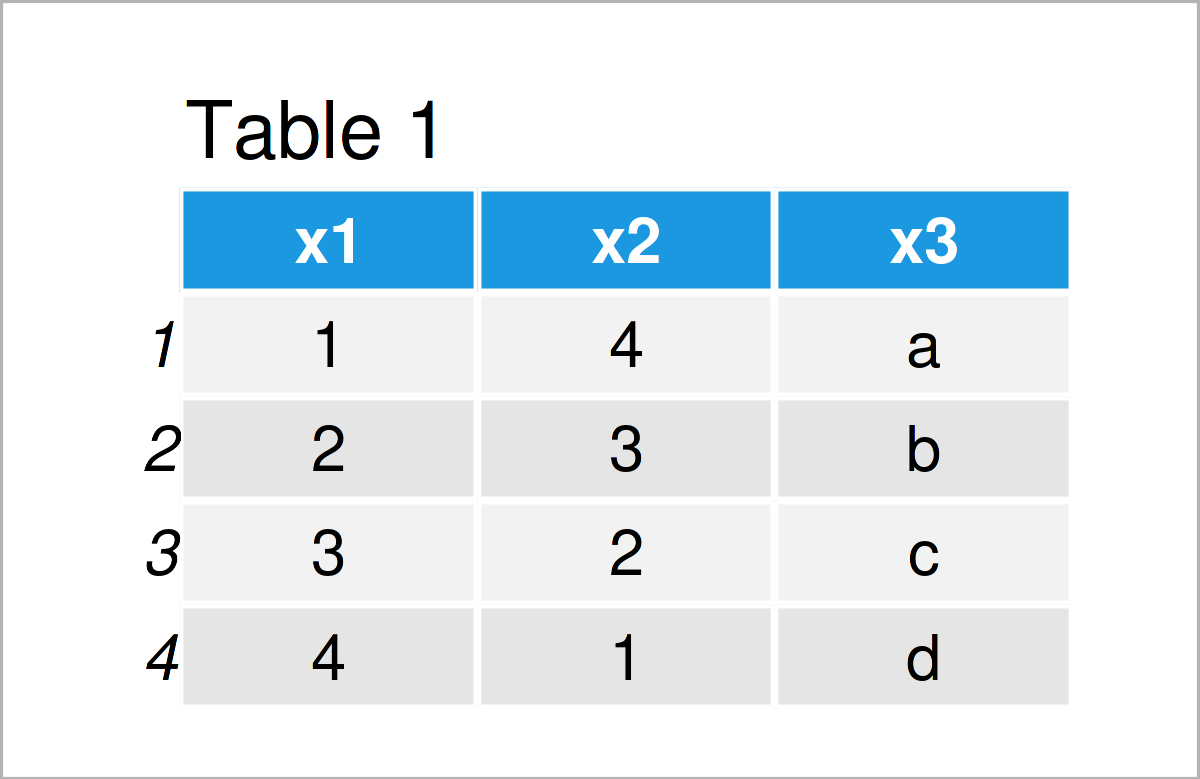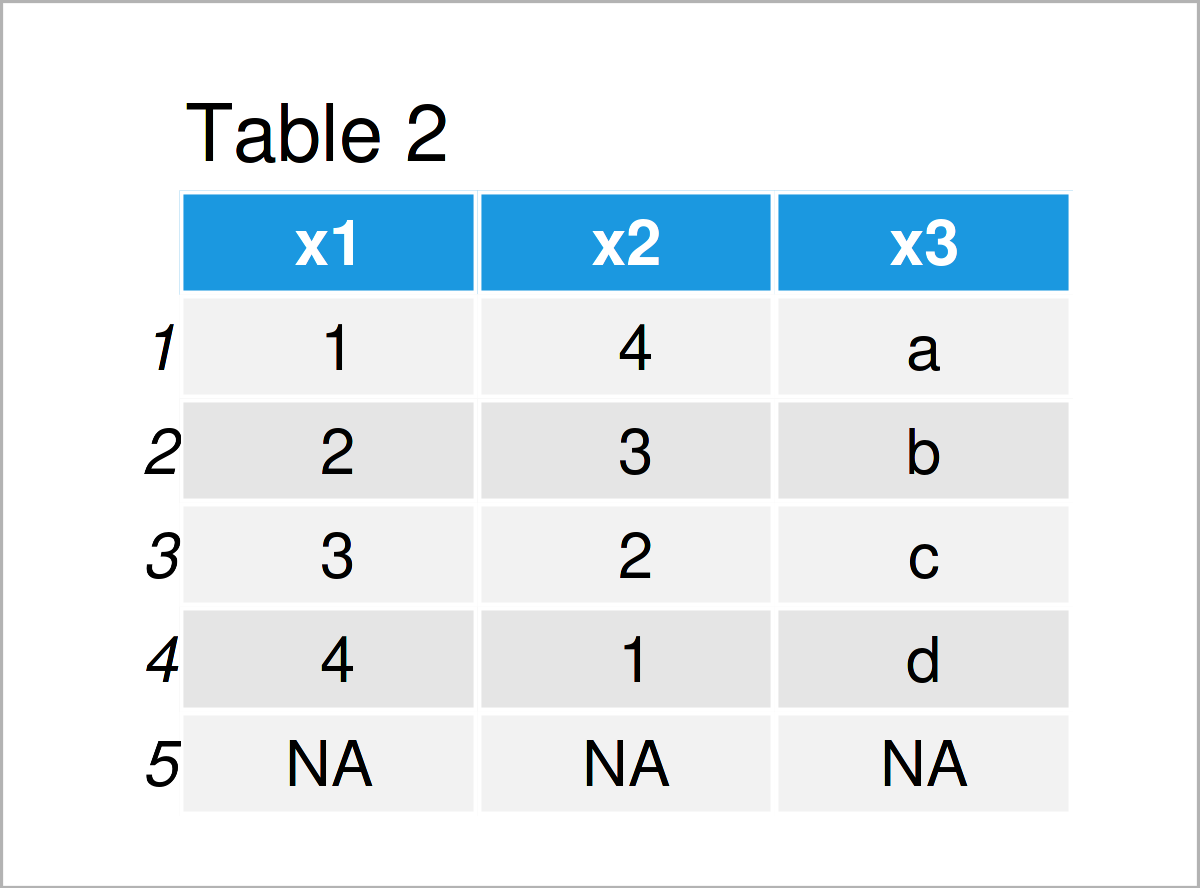# Add Empty Row to Data Frame in R (Example)

In this tutorial, I’ll explain how to append an empty row to the bottom of a data frame in the R programming language.

It’s time to dive into the example:

## Example Data

At the start, we’ll need to construct some example data:

```data <- data.frame(x1 = 1:4, # Create example data frame x2 = 4:1, x3 = letters[1:4]) data # Print example data frame```Have a look at the previously shown table. It shows that the example data frame is made up of four rows and three columns.

## Example: Append Empty Row to Data Frame Using nrow() Function

This example shows how to bind a new empty row that contains only NA values (i.e. missing values) to the bottom of a data frame object.

To accomplish this task, we can use the nrow function as shown in the following R programming syntax:

```data_new <- data # Create duplicate of example data frame data_new[nrow(data_new) + 1, ] <- NA # Add empty row containing only NA values data_new # Print new data frame```The output of the previous R syntax is shown in Table 2 – We have created a new data frame object called data_new that contains an additional line at the very bottom of the data frame. This new row contains only NA values.

## Video & Further Resources

Have a look at the following video on my YouTube channel. In the video, I’m illustrating the topics of this page.

In addition to the video, you might want to have a look at the related R articles on this website:

In this tutorial you have learned how to add an empty row with only NA values to the bottom of a data frame in the R programming language. In case you have additional questions, please let me know in the comments.

Subscribe to the Statistics Globe Newsletter